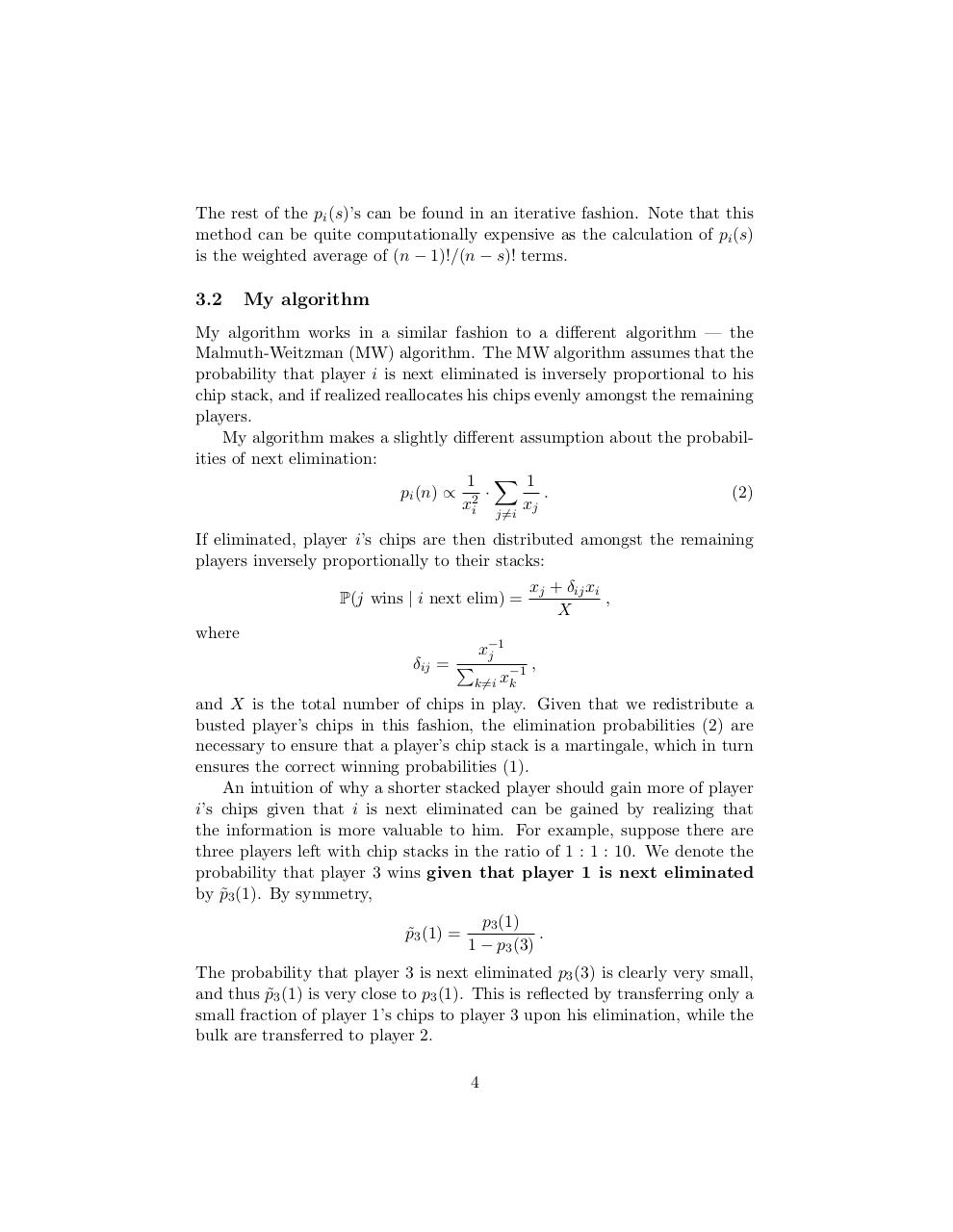# PDF Archive

Easily share your PDF documents with your contacts, on the Web and Social Networks.

## BenRobertsEquityModel.pdfPage 1 2 3 4 5 6 7 8 9 10

#### Text preview

The rest of the pi (s)’s can be found in an iterative fashion. Note that this
method can be quite computationally expensive as the calculation of pi (s)
is the weighted average of (n − 1)!/(n − s)! terms.

3.2

My algorithm

My algorithm works in a similar fashion to a different algorithm — the
Malmuth-Weitzman (MW) algorithm. The MW algorithm assumes that the
probability that player i is next eliminated is inversely proportional to his
chip stack, and if realized reallocates his chips evenly amongst the remaining
players.
My algorithm makes a slightly different assumption about the probabilities of next elimination:
1 X 1
pi (n) ∝ 2 ·
.
(2)
xj
xi
j6=i

If eliminated, player i’s chips are then distributed amongst the remaining
players inversely proportionally to their stacks:
P(j wins | i next elim) =
where
δij = P

x−1
j

−1
k6=i xk

xj + δij xi
,
X
,

and X is the total number of chips in play. Given that we redistribute a
busted player’s chips in this fashion, the elimination probabilities (2) are
necessary to ensure that a player’s chip stack is a martingale, which in turn
ensures the correct winning probabilities (1).
An intuition of why a shorter stacked player should gain more of player
i’s chips given that i is next eliminated can be gained by realizing that
the information is more valuable to him. For example, suppose there are
three players left with chip stacks in the ratio of 1 : 1 : 10. We denote the
probability that player 3 wins given that player 1 is next eliminated
by p˜3 (1). By symmetry,
p˜3 (1) =

p3 (1)
.
1 − p3 (3)

The probability that player 3 is next eliminated p3 (3) is clearly very small,
and thus p˜3 (1) is very close to p3 (1). This is reflected by transferring only a
small fraction of player 1’s chips to player 3 upon his elimination, while the
bulk are transferred to player 2.
4